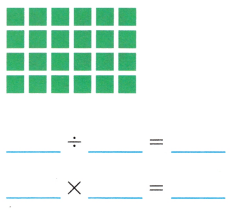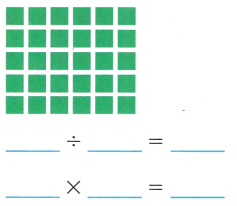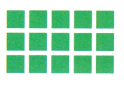Refer to our Texas Go Math Grade 3 Answer Key Pdf to score good marks in the exams. Test yourself by practicing the problems from Texas Go Math Grade 3 Unit 2 Assessment Answer Key.

Vocabulary

Choose the best term from the box.

Question 1.
An ___ is a number sentence that uses the equal sign to show that two numbers are equal. (p. 320)
Explanation:
An equation is a mathematical statement where the equal sign is used to show equivalence between a number or expression on one side of the equal sign to the number or expression on the other side of the equal sign.

Question 2.
___ have the same number of objects in each group. (p. 173)
Explanation:
equal groups– groups that have the same number of objects. For example, 5 x 6 = 30. There are 5 equal groups of 6 in 30.

Question 3.
When you place the saiiie number of tiles in each row, you make an ___. (p. 191)
Explanation:
You make an array by placing the same number of tiles in each row.

Question 4.
The ___ states that the product of zero and any number is zero. (p. 204)
Explanation:
The multiplication property states that the product of any number and zero is zero. It doesn’t matter what the number is, when you multiply it to zero, you get zero as the answer. So: 2 x 0 = 0.

Concepts and Skills

Write a division equation for the model. Then write a related multiplication sentence. TEKS 3.4.F., 3.4.J, 3.4.K, 3.5.B.

Question 5.Explanation:
That two equations are inverse multiplications.

Question 6.Explanation:
That two equations are inverse multiplications.

Question 7.Explanation:
That two equations are inverse multiplications.

Use properties to group the factors another way. Then find the product. TEKS 3.4.G

Question 8.
3 × (3 × 30)
Explanation:
3 × (3 × 30) = 3 X 90 = 270

Question 9.
(40 × 2) × 2
Explanation:
(40 × 2) × 2 = 80 X 2 = 160

Question 10.
3 × (50 × 1)
Explanation:
3 × (50 × 1) = 3 X 50 = 150

Question 11.
(60 × 3) × 2
Explanation:
(60 × 3) × 2 = 180X 2= 360

Texas Test Prep

Fill in the bubble for the correct answer choice. Use strategies you have learned to solve problems.

Question 12.
Vienna buys 6 boxes of crayons. If there are 8 crayons in each box, how many crayons does she have? TEKS 3.4.E, 3.4.K
(A) 48
(B) 14
(C) 32
(D) 12
Explanation:
6 boxes X 8 crayons = 48 crayons

Question 13.
The school center prints a newsletter that uses 6 pieces of paper. How many pieces of paper are needed to print 75 copies of the newsletter? TEKS 3.4.G
(A) 420
(B) 450
(C) 285
(D) 300
Explanation:
6 pieces of paper X 75 copies = 450 pieces of paper

Question 14.
Morgan has 6 toy cars. Each car has 4 wheels. How many wheels do the cars have in all? TEKS 3.4.E, 3.4.K(A) 2
(B) 10
(C) 24
(D) 12
Explanation:
6 toy cars X 4 wheels = 24 wheels

Question 15.
James made 2 arrays to show the Commutative Property of Multiplication. Which is a multiplication sentence for each array. TEKS 3.4.E, 3.4.K, 3.5.B(A) 3 × 5 = 15; 5 × 3 = 15
(B) 15 – 5 = 10; 15 – 3 = 12
(C) 2 × 3 = 6; 3 × 2 = 6
(D) 4 × 3 = 12; 3 × 4 = 12
Answer: (D) 4 × 3 = 12; 3 × 4 = 12
Explanation:
The sum and the product of two whole numbers remain the same even after interchanging the order of the numbers

Texas Test prep

Fill in the bubble for the correct answer choice.
Use strategies you have learned to solve problems.

Question 16.
Aidan bought 18 goldfish and 3 fish bowls. He put an equal number of fish in each fishbowl. Which equation can be used to find how many goldfish are in each fishbowl? TEKS 3.4.H, 3.4.K, 3.5.B
(A) 18 – 3 = __
(B) 3 + ___ = 18
(C) 18 ÷ 3 = __
(D) 3 × 18 = __
Answer: (C) 18 ÷ 3 = __
Explanation:
Aidan bought 18 goldfish and 3 fish bowls. He put an equal number of fish in each fishbowl.to find how many goldfish are in each fishbowl, we need to divide the 18 with 3.

Question 17.
James made an array with 6 rows of 4 blocks. Which number sentence shows one way to break apart his array to find the product? TEKS 3.4.K, 3.5.B(A) 6 × 4 = (6 × 4) + (6 × 4)
(B) 6 × 4 = (6 × 2) + (6 × 2)
(C) 6 × 4 = (6 + 2) + (6 + 2)
(D) 6 × 4 = (3 × 2) + (3 × 2)
Answer:  (B) 6 × 4 = (6 × 2) + (6 × 2)
Explanation:
You make an array by placing the same number of tiles in each row.
6 × 4 = (6 × 2) + (6 × 2)
24= 12 + 12
24=24

Question 18.
Derek has 6 dogs. Each dog gets 3 dog biscuits every day. How many biscuits will Dexter need for all of his dogs for Saturday and Sunday? TEKS 3.4.K
(A) 18
(B) 8
(C) 16
(D) 36
Derek has 6 dogs. Each dog gets 3 dog biscuits every day. So each day he need 6 dogs X 3 biscuits =18 biscuits.
Dexter need for all of his dogs for Saturday and Sunday (two days) is 36. Since,
6 dogs X 3 biscuits =18 biscuits. and for 2 days is 18 x 2= 36.

Question 19.
Which of the following multiplication equations can be used to find 63 ÷ 7? TEKS 3.4.F, 3.4.J. 3.4.K, 3.5.B
(A) 7 × 7 = 49
(B) 6 × 7 = 42
(C) 7 × 9 = 63
(D) 7 × 5 = 35
Answer: (C) 7 × 9 = 63
Explanation:
7 × 9 = 63  is the multiplication equations can be used to find 63 ÷ 7

Texas Test Prep

Fill in the bubble for the correct answer choice.

Question 20.
There are 54 students in the marching band. They form equal rows, so that there are no fewer than 5 rows and no more than 10 students in each row. Which is an array that would show how many rows they form and how many in each row? TEKS 3.4.F, 3.4.J, 3.4.K, 3.5.B
(A) 6 rows of 9 students
(B) 5 rows of 10 students
(C) 10 rows of 5 students
(D) 6 rows of 8 students
Answer:(A) 6 rows of 9 students
Explanation:
6 x 9 = 54,  so it is satisfying the no fewer than 5 rows and no more than 10 students in each row

Question 21.
There are 15 boys going camping. They brought 5 tents. An equal number of boys sleep in each tent. How many boys will sleep in each tent? TEKS 3.4.H, 3.4.K(A) 2
(B) 3
(C) 10
(D) 25
Explanation:
15 divided by 5 is 3

Question 22.
Cherokee’s apartment number is an even product between 30 and 40. One factor is even and one factor is odd Both factors are 1-digit numbers. What is his apartment number? TEKS 3.4.1
(A) 37
(B) 32
(C) 36
(D) 34